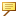Operators and Functions for Strings
The following operators and functions are supported for strings:
 == Compares strings as equal. !=, <>, ~= Compares strings as unequal. + Concatenates strings. itos(int) Converts integers to strings. Here, int can be a number or an expression. Nonintegers are rounded off. search(string, substring) Searches for substrings. The resulting value is the position of the substring in the string (0 if not found). You can specify substrings with single or double quotes. extract(string, position, length) Extracts pieces of strings. string_starts (string 1, string 2) TRUE, if the value of string 1 starts with the value of string 2. string_ends (string 1, string 2) TRUE, if the value of string 1 ends with the value of string 2. string_match (string 1, string 2) TRUE, if the value of string 1 matches the value of string 2.
For example:
If param = abcdef, then:
flag = param == abcdef—returns TRUE
flag = abcdef != ghi—returns TRUE
new = param + ghinew is abcdefghi
new = itos(10 + 7)new is 17
new = param + itos(1.5)new is abcdef2
where = search(param, bcd)where is 2
where = search(param, dcd)where is 0
new = extract(param,2,3)new is bcdIf you use the itos function on a parameter whose value is zero (0), the return value is an empty string.
The following examples illustrate the itos function:
integer_param = 4
string_param = itos(integer_param)
/*string_param will return 4 */
integer_param = -7
string_param = itos(int_param)
/*string_param will return -7 */
For an integer with zero (0) value, the itos function returns a null ("") value as shown below:
integer_param = 0
string_param = itos(int_param)
/*string_param will return an empty or null string ("") */
To return a zero string value ("0"), use the following IF statement:
integer_param = 0
string_param = itos(integer_param)
IF string_param == ""
string_param = "0"
ENDIF